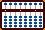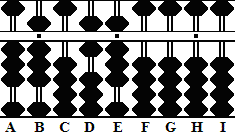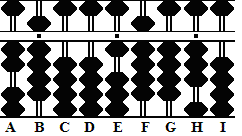###DIVISION REVISION EXAMPLES

The Bead Unbaffled - An Abacus Manual

When setting up problems of division, the abacus committee suggests leaving four empty rods in between divisor and dividend. Because space is limited, I'll have to bend the rules and leave only two.

As already pointed out, it's the ease with which quotient answers can be revised that makes a soroban such a wonderful tool for solving problems of division. In the following two examples I'll make mistakes, then quickly revise my wrong answers to put me back on the right track.

Example: 2584 ÷ 38  = ??

In this example, the dividend has four whole numbers. Choose E as the unit rod and count four rods to the left. The divisor has two whole number so count two plus two back to the right. This brings us back to rod E. Set the first number of the dividend on rod E.

Step 1: Set the dividend 2584 on rods EFGH and the divisor 38 on AB. (Fig.1)Fig.1 ```Step 1 A B C D E F G H I . . .   3 8 0 0 2 5 8 4 0 ```

Step 2:
Because the first two digits in the divisor are larger than those of the dividend, apply  Rule II Set the first number in the quotient one rod to the left of the dividend, in this case on rod D. Divide 3 on B into 25 on rods EF. Estimate a quotient of 7. Set the quotient 7 on rod D.

2a: Multiply the quotient 7 by the 3 on rod A and subtract the product 21 from 25 on rods EF.

2b: Multiply the quotient 7 by 8 on rod B and subtract the product from rods  FG.

**In order to continue there must be at least 56 on rods FG. There's only 48. The estimated quotient of 7 was too large.**

2c & 2d: Revise both the quotient and the dividend. Subtract 1 from 7 on rod D and add 3 to 4 on rod F. This leaves the revised quotient 6 on rod D and  the revised dividend 78 on rods FG. (Fig.2)

Step 2e: Now that we've revised the quotient, we can continue. Multiply the revised quotient 6 by 8 on B and subtract the product 48. This leaves the partial quotient 6 on rod D and the remainder 304 on rods FGH. (Fig.2)Fig.2 ```Step 2 A B C D E F G H I . . .   3 8 0 0 2 5 8 4 0 (7) Step 2 - 2 1 Step 2a 3 8 0 7 0 4 8 4 0 - ? ? Step 2b - 1 Revise (2c) + 3 Revise (2d) 3 8 0 6 0 7 8 4 0 (6) - 4 8 Step 2e 3 8 0 6 0 3 0 4 0 ```

Step 3: In looking at 38 on rods AB and 304 on rods FGH, it seems reasonable to estimate a quotient of 7. Set 7 on rod E.

Step 3a: Multiply the quotient 7 by 3 on A and subtract the product 21 from rods FG.

Step 3b: Multiply the quotient 7 by 8 on B and subtract 56 from rods GH

**With 38 remaining on rods GH, the estimated quotient of 7 was too small.**

3c & 3d & the answer: Revise both the quotient and the dividend. Add 1 to the quotient on rod E to make it 8. Subtract a further 38 from rods GH. This leaves the answer 68 on rods DE. (Fig.3)Fig.3 ```Step 3 A B C D E F G H I . . .   3 8 0 6 0 3 0 4 0 (7) Step 3 - 2 1 Step 3a 3 8 0 6 7 0 9 4 0 - 5 6 Step 3b 3 8 0 6 7 0 3 8 0 + 1 Revise (2c) - 3 8 Revise (2d) 3 8 0 6 8 0 0 0 0 ```

Example: 1943 ÷ 29  = ??

In this example, the dividend has four whole numbers. Choose rod E as the unit and count four rods to the left. The divisor has two whole number so count two plus two back to the right. This brings us to rod E. Set the first number of the dividend on rod E.

Step 1: Set the dividend 1943 on rods EFGH and the divisor 29 on AB. (Fig.4)Fig.4 ```Step 1 A B C D E F G H I . . .   2 9 0 0 1 9 4 3 0```

Step 2:
In this problem, the first two digits in the divisor are larger than those of the dividend. Therefore apply  Rule II.  Set the first number in the quotient one rod to the left of the dividend, in this case on rod D. Divide 2 on A into 19 on rods EF. Estimate a quotient of 7. Set the quotient 7 on rod D.

2a: Multiply the quotient 7 by the 2 on rod A and subtract the product 14 from 19 on rods EF.

2b: Multiply the quotient 7 by 9 on B and subtract the product from rods FG.

**In order to continue there must be at least 63 on rods FG. There's only 54. The estimated quotient of 7 was too large.**

2c & 2d: Revise both the quotient and the dividend. Subtract 1 from 7 on rod D and add 2 to 5 on rod F. This leaves the revised quotient 6 on D and the revised dividend 74 on rods FG. (Fig.2)

Step 2e: Now, continue with the revised quotient. Multiply the revised quotient 6 on D by 9 on B and subtract the product 54 from 74 on rods FG. This leaves the partial quotient 6 on rod D and the remainder 203 on rods FGH. (Fig.5)Fig.5 ```Step 2 A B C D E F G H I . . .   2 9 0 0 1 9 4 3 0 (7) Step 2 - 1 4 Step 2a 2 9 0 7 0 5 4 3 0 - ? ? Step 2b - 1 Revise (2c) + 2 Revise (2d) 2 9 0 6 0 7 4 3 0 (6) - 5 4 Step 2e 2 9 0 6 0 2 0 3 0 ```

Step 3: In looking at 29 on rods AB and 203 on FGH, it seems reasonable to estimate a quotient of 8. Set 8 on rod E.

Step 3a: Multiply the quotient 8 by 2 on A and subtract the product 16 from rods FG.

Step 3b: Multiply the quotient 8 by 9 on B and subtract the product from rods GH

**In order to continue there must be at least 72 on rods GH. There's only 43. The estimated quotient of 8 was too large.**

3c & 3d: Revise both the quotient and the dividend. Subtract 1 from 8 on rod E and add 2 to rod G. This leaves the revised quotient 7 on rod D and  the revised dividend 63 on rods GH.

Step 3e & the answer: Now that we've revised the quotient, we can continue. Multiply the revised quotient 7 by 9 on B and subtract the product 63. This leaves the answer 67 on rods DE. (Fig.6)Fig.6 ```Step 3 A B C D E F G H I . . .   2 9 0 6 0 2 0 3 0 (8) Step 3 - 1 6 Step 3a 2 9 0 6 8 0 4 3 0 - ? ? Step 3b - 1 Revise (3c) + 2 Revise (3d) 2 9 0 6 7 0 6 3 0 (7) - 6 3 Step 2e 2 9 0 6 7 0 0 0 0```

 Here's a link to two more examples: 35405 ÷ 97 = 365 278502 ÷ 399 = 698

Back to Abacus: Mystery of the Bead

Print Page (.pdf format,127kb)